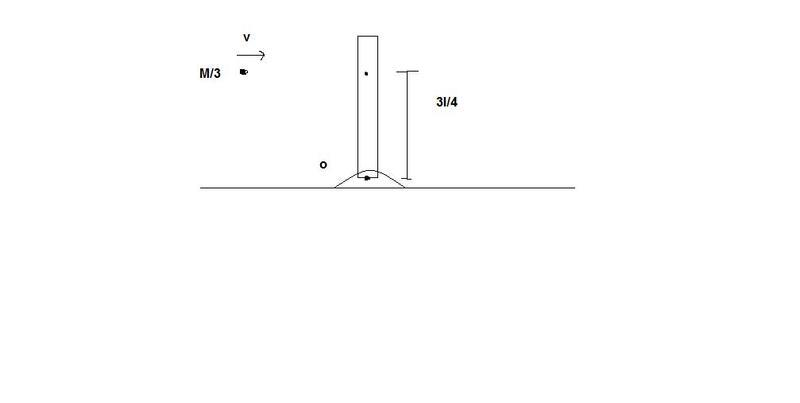# Rigid Body Collision Problem

• zorro
Ok, so the centripetal force is just the weight of the rod multiplied by ω2?Yep, that's it.The centripetal force is just the weight of the rod multiplied by ω2.

## Homework Statement

A uniform rod of mass M and length l is hinged at point O as shown in fig. A small particle of mass M/3 strikes the rod with velocity v at a distance 3l/4 from O and sticks to it. Find the value of v such that the force exerted by the hinge on the rod just after the impact is 0.## The Attempt at a Solution

There are two forces exerted by the hinge, namely normal force and force due to impulse.
What will be the direction of the force due to impulse? Which force should be 0 according to the question?

#### Attachments

Hi Abdul!(btw, please try to post images that fit the usual frame width)

Use conservation of angular momentum about the pivot to find the initial angular velocity.

Then use conservation of angular momentum about the combined centre of mass to find the initial torque at the pivot (and equate it to zero) …

what do you get?What will be the direction of the force due to impulse?

what is the direction of acceleration of the centre of mass?tiny-tim said:
Hi Abdul!(btw, please try to post images that fit the usual frame width)

Use conservation of angular momentum about the pivot to find the initial angular velocity.

I did that before postingtiny-tim said:
Then use conservation of angular momentum about the combined centre of mass to find the initial torque at the pivot (and equate it to zero) …

Torque = F*9l/16, where F is the force exerted at the hinge/pivot.
Equating it to 0 gives F=0. What next?

hmm … I didn't put that very well last time… I should have said:

Use conservation of angular momentum (about the combined centre of mass) …

if the impulsive torque is zero, then you know that the angular momentum will be conserved!tiny-tim said:
Use conservation of angular momentum (about the combined centre of mass) …

if the impulsive torque is zero, then you know that the angular momentum will be conserved!Use conservation of angular momentum ( about the combined centre of mass )...angular momentum will be conserved.

Both imply the same thing. I don't get you.

Both imply the same thing.

Yes, the second is only there to explain to you why the first works.Ok...
By conserving the angular momentum about the combined centre of mass,

M/3*v*(3l/4 - 9l/16) = Icombined C.O.M.*wcombined C.O.M.

where ω=12v/25l (which you suggested to find in your first post)

But the 'v' cancels out!

Hi Abdul!Yes, you're right … the v cancels out.Ahh, I've completely misunderstood the questionit isn't saying that the impulsive force during the collision is zero, it's saying that the ordinary force immediately after the collision is zero …

so (complete change of plan) you need to find the total centripetal force on the system (as a multiple of ω2), and compare it with the gravitational force …

then the force at the pivot will be zero if … ?tiny-tim said:
it's saying that the ordinary force immediately after the collision is zero …

Ordinary force = ?
The normal force by the pivot? If it is 0 then the rod will fly away!

No, gravity will hold it there temporarily.What about the tension in the rod?

What tension?Get on with it!

Tension due to the impulsive force at the pivot - which gives the rod a jerk.